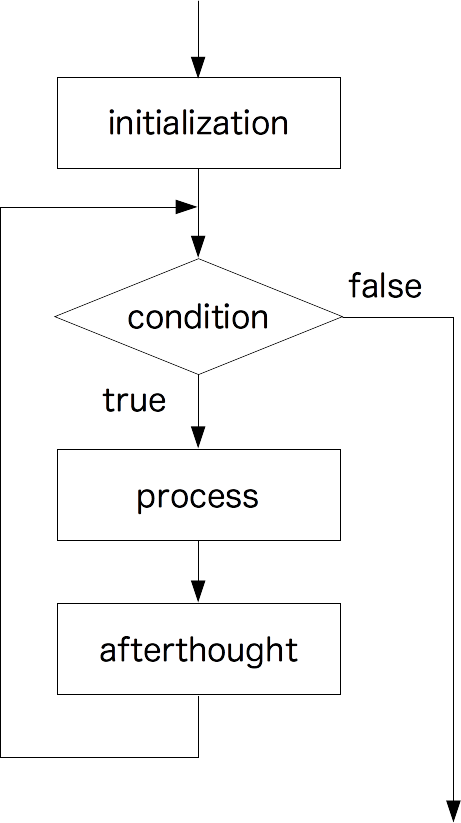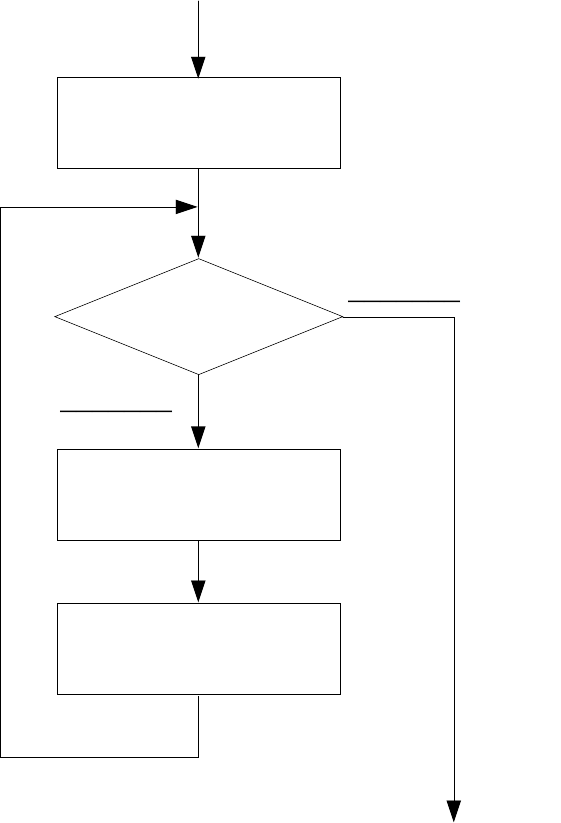# No.9 Loop

## The Change of Values in a Variable

### Calculation using the Current Value

Data can be stored into variables by assignment.When a result of some formula is stored into a varible, the “current value” of the variable can be used in the formula. For example, the following is an assignment statement for a variable `x`.

• `x = x + 1;`

If the original value of x is 2, first calculate `x + 1` which results 3, and then 3 is assigned to `x`. So after the statement is executed, the value of `x` will change to 3.

Exercise 09-1（☆）
1. Execute the following with console.
• `var x = 3;`
• `x = x + 1;`
• `x = x + 1;`
• `x = x + 1;`
2. Execute the following with console.
• `var x = 1;`
• `x = x * 2;`
• `x = x * 2;`
• `x = x * 2;`
• `x = x * 2;`

When writing statements like the example above, it is a waste of time to write the same variable twice.  Instead, the operator `+=, -=, *=, /=` … can be used.  For instance, x = x + 1 can be written as x +=1, or x = x – 1 can be written as x -=1.  There is a special operator for “adding 1″(`++x`) and “subtracting 1″(`--x`).  All of `x = x + 1, x += 1, ++x` have the same meaning. `x++` is also an operator for “adding 1”, but has a slightly different meaning (see the reference below).

### How to use the Debugger

A debugger can be used when we want to know which part of the program is running, or what value is inside a variable.

The following can be done with a debugger.

Pausing a Program, Step-by-step Execution, Resume
The program can be stopped by setting a breakpoint.  You can resume the program or execute the program step-by-step.
Displaying/Changing the Value of a Variable
The variable can be displayed/changed when the program is paused at a breakpoint.
Exercise 09-2（☆☆☆）
1. Make `ex09-2.html` and fill in the following.
2. Make `ex09-2.js` and fill in the following.
3. Click the button several times.  What happened to the number?
4. Watch the video below while doing the following steps.
5. Open `ex09-2.html `via Firefox, and select Tools→Web Developer→Debugger
6. The left section shows the files used in the webpage,  and the JavaScript program will show up when you click `ex09-2.js.`
7. A breakpoint can be set by clicking on the number of the line you want to stop.  Click on the 5th line and make a breakpoint right before `alert`.
8. Click on the “execute” button(on the webpage, not debugger), and call out `addCount(). `What change on the debugger screen?
9. The value of variables are displayed on the right section of the debugger.  “Scope” is a little difficult and won’t be explained in this class, but when a variable is declared inside a funtion, it is a funtion scope, and when a variale is declared outside any funtion, it is called a global scope.  Click on the triangle next to “Global Scope” and check the value of `count`.
10. Clicking on the triangle above the file list will resume the program execution.  Resume the program and check that the value of `count `and the number displayed on the dialog box are equal.
11. Click on the 4th line and make a break point before `count = count + 1`.
12. Click on the “execute” button to call out `addCount(), `and check the value of count.
13. Resume the program.  It will stop again at the 5th, so check the value of  `count`.
14. Resume the program and check what number is displayed in the dialog.
15. You can turn off the break point by clicking.  Turn off the break point on the 4th and 5th line.

Reference

### Changing the Elements of HTML

In Exercise 08-8, the width of the image was set to 800 when the button was clicked. Let’s change the function so that the width gets 10 pixels larger each time the button is clicked.

Exercise 09-3（☆☆☆）
1. Make `ex09-3.html` and fill in the following.
2. Make `ex09-3.js` and fill in the following.
3. Open the file via browser and click the button.
4. Declare a variable `w` on the top of `ex09-3.js` and assign the value to 100.
5. In `setAttribute`, change the width size from the number `'800'` to the variable `w`.
6. In function `change`, write a statement “add 10 to the variable `w`” and change the function so that the image gets larger each time the button is clicked.
Exercise 09-4（☆☆☆☆）

Make a textbox, and add a function so that when a number is entered, the image gets larger by the value of the number.

### Adding Content to a Page

In Exercise06-8, we learned that the content of the webpage can be changed by using `innerHTML`. By assigning different values to `innerHTML` again and again, we can gradually change the content of a webpage.

Exercise 09-5（☆☆☆）
1. Make `ex09-5.html` and fill in the following.

2. Make `ex09-5.js `and fill in the following.
3. Open the file via browser and click the button.  What happened?
4. On the 2nd and 3rd line of `ex09-5.js`, what was assigned to variable x?
5. What happened to the structure (the relationship of `ul` and `li`) of the HTML?
6. Click the button several times.  Did the number of cookies increase?
7. Make a variable `b` at the top of `ex09-5.js` to store the number of cookies.  Assign 1 for its initial value.
8. Change “there’s one cookie” to “there are n cookies”. The value of variable `b` should go into the place of “n”.
9. Add a statement in `knock()` that adds 1 to variable `b`.
10. Reload the page, click the button and check if the number of cookies increase.

## Automatic Loops

In the exercise above, we must click the button multiple times to repeat the process.  Let’s try repeating the process several times with one click.

### “for” Loops

To repeat processes. we use the “for” loop.  A for loop is written as the following.

• `for ( initialization; conditoin; afterthought ) { process }`

This statement will be executed in the following way.

1. The “initialization” part is executed.
2. The “conditon” part is calculated.
3. If the condition is true, the “process” part is executed.
4. The “afterthought” part is executed.
5. The “conditon” part is calculated again. If it is true, the “process” and “afterthought” parts are executed again.
6. The step above is repeated while the condition is true. When the condition is false, we go to the next statement.Exercise 09-6（☆☆☆）
1. Make `ex09-6.html` and fill in the following.
2. Make `ex09-6.js` and fill in the following.
3. Open the file and click the button.
4. Draw the following diagram on your notebook. Fill its blank boxes with sentences in `ex09-6.js`.5. Replace `count <= 5` in the second line with `count >= 5`, reload, and click the button. Why the behavior changes like this?
6. Replace it with `count >= 0`, reload, and click the button. Why the behavior changes like this?
Exercise 09-7（☆☆☆☆）

Edit Exercise09-5 so that 100 lines are added at once when the button is clicked.

Exercise 09-8（☆☆☆☆）

Make a textbox, and add a function which repeats the process until the number of cookies reaches the input number.

You may think the image file in Exercise 09-3 would get larger and larger if using a for loop, but it won't work.  The repetition of the for loop is done in an instant, so to the naked eye it would seem as if only the last photo is displayed.  The proper way to do this will be explained in Appendix: Various Features - Iteration by Timer Events.

### "while" Loops and "do-while" Loops

"while" loops and "do-while" loops can be used for loops as well.  We can do the same thing with any of them, so use the loop you are most comfortable with.

"while" loops are written as the following.

• `while ( condition ) { process }`

"do-while" loops are written as the following.

• `do { process } while ( condition )`

The process is executed repeatedly while the condition is true.  With a "while" loop, if the condition is false in the first place, the process isn't executed at all.  With a "do-while" loop, the process will be executed at least once.

The "for" loop has the same meaning with the following "while" loop.

1. `initialization; while ( condition ) { process; afterthought; }`
Exercise 09-9（☆☆☆☆）

Rewrite the program with while loops, for Exercise 09-6 and Exercise 09-7.

Points to check
• What does `x = x * 2 `mean?
• How is a for loop written?
• What happens when the condition is false after initialization of a for loop?